Reach Us+44-1522-440391
A Robust Method for Fingerprint Recognition using Biometric Fusion | OMICS International
Journal of Biometrics & Biostatistics

Like us on:

All submissions of the EM system will be redirected to Online Manuscript Submission System. Authors are requested to submit articles directly to Online Manuscript Submission System of respective journal.

# A Robust Method for Fingerprint Recognition using Biometric Fusion

Nasrollah Moghadam, Mehdi Abadi and Hamza Ali Mahfoodh*

Department of Computer and Electrics Science, Faculty of Computer Engineering, TMU University, India

*Corresponding Author:
Hamza ali mahfoodh
Department of Computer andElectrics Science
Faculty of Computer Engineering
TMU University, India
E-mail: [email protected]

Received date: May 02, 2012; Accepted date: May 24, 2012; Published date: May 25, 2012

Citation:Moghadam N, Abadi M, Mahfoodh HA (2012) A Robust Method for Fingerprint Recognition Using Biometric Fusion. J Biom Biostat 3:143. doi:10.4172/2155-6180.1000143

Copyright: © 2012 Moghadam N, et al. This is an open-access article distributed under the terms of the Creative Commons Attribution License, which permits unrestricted use, distribution, and reproduction in any medium, provided the original author and source are credited.

Visit for more related articles at Journal of Biometrics & Biostatistics

#### Abstract

In this paper we will introduce a combined approach that uses the local and global features on the fingerprint. Minutiae features are extracted then a mesh of minutiae points relations will created. Then among the outputs of first stage two points used in the other stage as reference point and rotation handle. Matching process will take place after each stage then results made in both stages will be used to get the final answer. In this approach we used 10 fingerprints of persons to maximize the precision. Also we will use powerful pre-process tools to ensure that the input image is in the best condition for matching.

#### Introduction

Biometric systems operate on behavioral and physiological biometric data to identify a person. The behavioral biometric parameters are signature, gait, speech and keystroke, these parameters change with age and environment. However physiological characteristics such as face, fingerprint, palm print and iris remains unchanged throughout the life time of a person. The biometric system operates based on one of the verification or identification modes. In the verification mode the point is to match captured features of a person with one template to give one answer, yes or no. The identification mode recognizes the person by matching his biometric against every template in the database.

Because the simplicity and cost effectiveness of physiological biometrics they are first choice of any developer. in the other hand fingerprint between the physiological biometrics is the popular and most used one due its feasibility, distinctiveness, permanence, accuracy, reliability, and acceptability. Fingerprint matching systems can be categorized within two categories, Correlation based approaches and minutiae based approaches. In correlation based approaches the similarity of pixels is estimated. First step in this type is finding a singular point or two; by singular point we mean fingerprint core and delta. They use singular points as images reference point and rotation handle. If the fingerprint image contains only one singular point, it must be rotated in several angles to determine the best matching degree. Sir Henry in 1900 classified the fingerprints into five classes, Arch, tented arch, Whorl, Right loop and Left loop. In arch types there is no core point, this will be a problem when using correlation based approaches. In the other side Minutiae based approaches are more popular. This approaches use some local features on fingerprint ridges called minutiae points. Based on ridges shapes and their collisions the minutiae points called ridge endings, bifurcations, cross points and spurs and etc. the most common features used are endings and bifurcations. These features can be extracted from the thinned ridge image (Figure 1). Deciding what type is the feature, is based on nine neighbors of a point, so if there is any remains of noise in the image there will be a wrong feature.

It is difficult to extract reliably minutia from poor quality fingerprint impressions arising from very dry fingers and fingers mutilated by scars, scratches due to accidents, injuries.

Biometrics alone cannot provide sufficient accuracy to prevent fraud. Behavioral biometrics can be imitated and physiological biometrics can be fabricated. For example fingerprints are subject to forging using latex moulds or exemplary methods. Here comes biometric fusion as a solution. The biometric fusion term refers to engage more than one biometric in the process to enhance the accuracy. Generally biometric fusion can be performed in one of these forms: multiple sensors, algorithms, instances, samples, biometrics and hybrid. Note that the fusion can take place prior to match or after match (Figure 2).

The motivation behind this work is to introduce an approach which uses both correlation and minutiae based concepts for enhance both approaches and minimize their weaknesses.

This paper contains the following sections. Section II is an overview of the related work, in section III introducing our proposed method in detail. Section IV performance analysis and results are discussed, in section V gave the conclusions.

#### Related Work

The need of more accurate security systems derives developers to spend more time on accuracy enhancement researches. Human biometric are sometimes easy to fabricate, but when we combine multiple samples of a biometric or multiple biometrics, it will be very hard or impossible to forge them. Recently many researchers have looked at this issue .

Marcialis and Roli  used multi sensor fingerprint image and extracted each image features alone then fused the results to a single result. They used optical and capacitive image, because of their low price and easy to find properties. Again Marcialis and Roli  proposed the use of a perceptron with a class-separation loss function for combining multiple fingerprint matchers. Jain et al.  present a scheme to integrate the output scores of three different fingerprint matchers to improve the performance of a fingerprint verification system. Jain et al.  presented a fingerprint matching scheme that utilizes both the minutiae and texture information available in the fingerprint. A bank of Gabor filters is used to extract features from the tessellated cells of the template and input images. Although non-linear deformations present in the fingerprint images have not been accounted for by their method. Marcialis and Roli  fused two fingerprints in three modes using multiple algorithm fusion. Prabhakar and Jain  represented a decision level fingerprint fusion. Their design emphasis is on classifier selection before arriving at the final combination. It was shown that one of the fingerprint matchers in the given pool of matchers is redundant and no performance improvement is achieved by utilizing this matcher. Sha et al.  proposed a fingerprint matching technique that combines minutiae information with the ridge flow information. Ross et al.  propose a two-stage fusion scheme that takes advantage of the information among multiple fingerprint impressions. While comparing the query fingerprint with a template impression, the other impressions are also transformed using the 2D warping model to register with the query fingerprint so that the additive matched minutiae pairs can be detected. Then a matching score level fusion or decision level fusion is performed to integrate the matching results corresponding to different impressions (Table 1).

Accuracy Proc. time Storage
DB#1 99.3 1.327 ~25kb
DB#2 97.1 1.412 ~25kb
DB#3 93.0 1.602 ~24kb
DB#4 82.4 1.698 ~24kb
DB#5 63.9 1.751 ~23kb
Average 87.14 1.558 ~23kb

Table 1: Below you can see minutiae matching results in mentioned db’s.

#### Proposed Algorithm

In this section we’ll introduce our proposed algorithm in detail. Figure 3 gives the block diagram of our proposed algorithm.

It is a critical step to prepare the fingerprint image before processing step. The image that scanned and stored, may suffer from several problems. Noise on the fingerprint image usually makes fake features. Cut lines are another example of fingerprints faults that may produce wrong features among feature extraction. In the other hand many fingerprint images suffer from low quality because the user doesn’t push his finger enough on the sensor, or pushing too much. So plus to a strong pre-processing module we need several rules to prevent wrong features show up in the feature vector.

After image acquisition, the grey-scale fingerprint converted to black white image. Generally this step is performed by thresholding methods. But we did this step a little different. By averaging the minimum and maximum colors on image with 9 points on the image, we made our threshold and then every pixel below threshold value converted to black and every pixel above the threshold converted to white.

Second, the resulting image has to be segmented therefore the image must get normalized in other words fingerprint ridges intensity standard deviation on the image must be equal to zero. Then by subdividing the image into 16 fixed size blocks, the standard deviation calculated for each block. If the resulting number is above the defined threshold (0.1-0.2) then the area considered a part of the fingerprint. Figure 4 shows areas that are suspect to be ridges on a given fingerprint. Note that the before fingerprint has passed the previous steps (Table 2).

Core Detection (s) Accepted CP (%)
Proposed approach --- 95.1
tomohiko's  0.365 96.0
navrit Kaur 's  0.68 85.6
ohtsuka's  0.44 90.8
kazuhiko's  0.641 93.0
Takeshi's  0.45 94.4
Atipat’s  0.548 77.0

Table 2: The correlation step results, you can see proposed algorithm does not take any time to align query and template images, while other algorithms spend a short time to calculate the core and delta points on the fingerprints.

Then the segmented ridges orientation must be estimated and a weight must be attached to every ridge parts. This step helps by looking at every local area. Ridges with a very different orientation got very low weights. Another advantage of this step is to connect ridges that got disconnected in acquisition step.

Local ridge orientation at p[x,y] is the angle θxy, that the ridges, crossing through an arbitrary small neighborhood centered at [x,y], form with the horizontal axis. Instead of computing local ridge orientation at each pixel, like most methods we estimate the local ridge orientation at discrete positions (see Figure 5).

Figure 5: Computing ridge orientations using 16*16 grid mesh. ϒ, refers to reliability of a local ridge. This value is low for noisy and damaged areas and high for good quality regions.

For estimating the ridges local orientations, we used gradient based approach , enhanced by kas and witkin. By doubling the angle they fixed the discontinuity and non-linearity around 90 degrees. So each orientation estimate is encoded by the vector: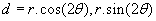The theta doubled to reduce of the circularity of angles and r is proportional to the orientation estimate strength. Averaging the angles in a local n*n window (w) to obtain more robust estimate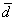, can be performed by averaging x and y separately.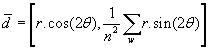Next the local ridge frequency must be calculated. A frequency image is analogues to orientation image. The goal of this stage is to calculate the number of the ridges in a defined area. This frequency varies between different fingerprints and differs between different areas of the same fingerprint.

To perform this step, an oriented m*n window centered on [x,y] (from the ridge orientation image), aligned within ridges orientation. The x-signature of the grey-level is obtained by accumulating. Distances between ridges that fell into the oriented window are averaged to obtain the ridge frequency. Figure 6 shows that how the x-signature and ridge distances produce ridge frequency. Figure 7 shows the frequency image made of grey-scale image.

Figure 6: Oriented window centered on [x, y] from orientation image, x-signature of the window, distances between the x-signature peaks and fij as the resulting local ridge frequency.

Figure 7: grey-scale image (a) versus frequency image(b) and polarized image(c). The ridges can easily recognized after applying past operations. Note that unreliable areas like nether the image removed from the resulting image due reliability measurement stage.

After calculating the local ridge frequency, a polarization operation must be performed on the image in order to enhancement. Polarization was introduced by Louis malls in 1800. Electromagnetic waves like light beam are constrained to vibrate in a certain plane or planes. Electromagnetic waves consist of vibrating electric and magnetic fields, with the direction of vibration perpendicular to the direction of motion of the wave. If the direction of vibration remains steady with time, the wave is said to be 100% linearly polarized in that direction. If the direction of vibration rotates at the same frequency as the wave, the wave is said to be 100% circularly polarized. Most naturally occurring electromagnetic waves have a direction of vibration that jiggles around at random: these are said to be un-polarized. Polarized imaged can be achieved by using two operators. First operator determines filtering bandwidth by controlling the image width sigma, second operator defines the filter orientation by controlling image length sigma. Figure 7 shows an image before and after applying polarization.

After preparing the grey-scale image, it is time to take the resulting image to feature extraction process, but before that, the approximate fingerprint rotation must be calculated, hence a filled fingerprint mask is used to specify the finger angle. As you know generally fingerprints surrounding shape is elliptical. An ellipse contains two diameters, transverse diameter and conjugate diameter, which are orthogonal to each other. Hence a fingerprint image rotation angle can be estimated by its transverse diameter, since it is like an ellipse. Note that this rotation estimation is elementary operation to help fingerprints get a semi-normal form before feature extraction. Figure 8 shows this mask on a given fingerprint.

After aligning the fingerprint in respect to the mask ellipse diameters, it remains in the memory, because this mask will be Participate in feature selection step. Next the image goes to feature extraction module.

In this step two common types of minutiae are considered as features, ridge endings and bifurcations. Therefore the image converted to thinned image, then after obtain cleaning process across the image, a 3 × 3 window surf the image and based on every non zero pixel and it’s eight neighbors, the minutiae points extracted based on this rules.

1. Any pixel with only one neighbor is ridge ending (RE).

2. Any pixel with 2 neighbors is a ridge passing point, so leave it.

3. Any pixel with 3 neighbors is bifurcation (BP) only if the neighbors are not side by side neighbors to each other.

Using these conditions the feature selection process doesn’t involve bifurcation validation measurement. One of the feature selection terms is, if a ending point located on a short line (for example shorter than 10 pixels), then avoid it. Another important feature selection term is that remove any ridge ending located on fingerprint surrounding. Here comes the fingerprint shape mask as a helpful tool. Any ridge ending correspond to the mask surrounding have to be removed. Bifurcations in those areas remain without change, because it is very odd to have fake bifurcation there. Resulting minutiae points are the features extracted from the fingerprint image. Then three meshes are created, one for RE-RE relations, one for BP-BP relations and another for RE-BP relations. By relations we mean connecting links. So for every minutiae all it’s relations represented by a connecting link (see Figure 9).

Figure 9: Ridge endings relations(a), Bifurcation relations(b), Ridge ending vs. Bifurcation relations.

The produced meshes can be described as nodes and weighted edges. By weight we mean distances between any vertexes to another. The distance between point’s p and q can be calculated using this equation: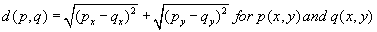So if we want to calculate this distance in polar coordinates we have: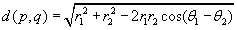Which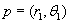and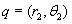in polar coordinates.

Both distances can be used, but the polar coordinates are used in a “good fingerprint rotation aligned”. This distance is one of the features, another feature can be derived from mesh edges, the angle between connecting links of one vertex. A Euclidean angle is completely determined by the corresponding right triangle. In particular, if θ is a Euclidean angle, then using trigonometric functions we find: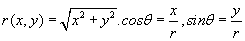for two numbers x and y.

So an angle in the Euclidean plane can be legitimately given by two numbers x and y. To the ratio y/x there correspond two angles in the geometric range 0 < θ < 2π, since: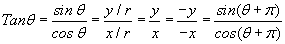If infinities are permitted for the quotient y/x one can define the angle θ as a function of x and y using the inverse tangent function for all points except the origin, assuming the inverse tangent varies from -π/2 to π/2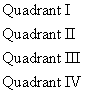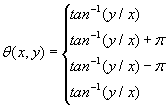Note that having the vertices all this relations can be retrieved, so there is no need to entering these relations within the feature vector. This will increase the processing time while matching two fingerprints, so if the storage capacity is enough, saving these features in the feature vector seems to be a better choice. So for any feature f, the feature vector is (type, relations to others, edges angle). But this process will take so long since any minutiae has n-1 connections to other minutiae points. Then we will have n×(n-1) weights and n×(n-1) angles. So we need a region of interest (ROI) to reduce these relations. The ROI area defined as a circular region around every minutiae point, with specific diameter, so that the minutiae is in relationship with another one if that point fell within the ROI, else the relation set to be infinity length. The diameter cannot be very short, because all the connections between one minutiae to other minutiae will be considered as infinity distances.

The matching process involves the features we discussed about. A threshold (t) is used to limit the fault tolerance. A distance measure (d) is used to determine the un-similarity between each pair fingerprints and pair of most similar minutiae points in corresponding fingerprints is selected for input image alignment purpose.

Next the query image is matched to the template image by correlation methods. This stage works as follows:

The query image placed on the template image using the most similar pair of minutiae points, resulted from minutiae matching stage. One of the minutiae points used to center both images on a single point. Second minutiae used to handle the query image rotation angle (Figure 10).

Figure 10: pair of most similar minutiae points (a), cropped area forwarded to next stage (b).

Having the connection between two most similar minutia points, we can calculate the final image rotation angle. The similarity then must be calculated by subtracting template image from query image. Remaining pixels considered as non-similarity ratio.

An iterative approach is used to minimize the 2D correlation coefficient by using nonlinear optimization techniques. The cross correlation coefficient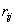defined as: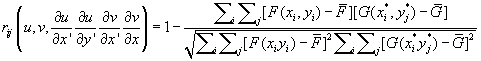Here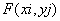is the pixel intensity or the gray scale value at a point (xi, yj) in the undeformed image.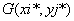is the gray scale value at a point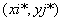in the deformed image.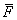and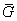Gare mean values of the intensity matrices F and G, respectively. The coordinates or grid points (xi, yj) andare related by the deformation that occurs between the two images. If the motion is perpendicular to the optical axis of the camera, then the relation between (xi, yj) andcan be approximated by a 2D affine transformation such as: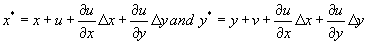Here u and v are translations of the center of the sub-image in the X and Y directions, respectively. The distances from the center of the sub-image to the point (x,y) are denoted by Δx and Δy. Thus, the correlation coefficient rij is a function of displacement components (u,v) and displacement gradients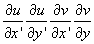Now the query image and the template image are placed on each other with minimum correlation coefficient (see Figure 11). Next the matching scores resulted from first stage and second stage must be combined to achieve final answer.

Figure 11: query and template fingerprints(a),(b), two points as best matches for alignment(c), image aligned and cropped then correlated according to maximum common area in both images(d).

For query image Iq and template image It , let Mq denotes minutiae points matched successfully and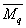denotes minutiae points not matched, and d denotes the distance (or weight) of matched minutiae points, and Cq regions are matched in second step of matching, then we have: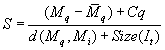So for n fingerprints we have: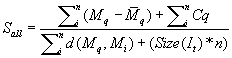Sometimes scores outputs by different matchers are homogenous, for example if two fingers of a person are being combined, it’s very likely that the scores do not need to be normalized. In case different fingers of a person are not equally distinctive, it may be desirable to normalize the scores, but generally these scores do not need normalization. But when different matching algorithms are used, even on the same finger, the scores are not homogenous. So these scores need to be normalized. Given a set of scores {sk}, k=1,2,...,n the normalized scores are given by: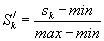where the minimum and maximum values are estimated from training data.

Suppose that we normalized the scores such that they Sj ∈[0,100], the combined score can be calculated by several methods, sum, product, maximum are examples of a fusion rule. But according to Maltoni et al.  showed that all these rules are special cases of a unified framework based on Bayesian decision theory. So for example if the scores are normalized such that Sj ∈[0,1], the score itself can be considered as an estimate of the posteriori probability of a genuine match, that is: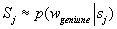We used the sum rule because product rule is very sensitive to errors. The sum rule can be used when the match scores tend to be noisy. We can assume that the posteriori probabilities are noisy. In such a case we can assume that the posteriori probabilities do not deviates significantly from the prior probabilities.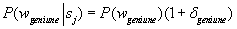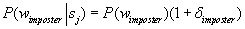where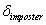,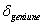are small deviations (<<1), j=1,2,…,R. assuming equal priors, and non-zero loss function. The sum rule can be stated as: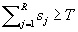where T is the threshold value.

#### Experimental Results

The proposed algorithm performance was tested on 1.6 GHZ processor with 512kb cache, 1GB ram, Windows XP, using matlab7.0.

The data base used is made of 880 fingerprints, scanned from 44 persons, 10 fingers per person and twice a finger. According to quality of the images we divided the fingerprints to 5 classes. First two classes contain fine fingerprints, with good quality. Third class contains fingerprints that are noisy and not full-print images. Fourth and fifth classes contain fingerprints with much of noise and bad quality due fingers dirt, dryness and other issues (Figure 12,13,14).

Figure 12: five classes of fingerprints, from good quality and noise less to noisy low quality images.

Figure 13: query images from db#4 (a) and db#3 (c), template images (b),(d), the matcher recognizes the corresponding fingerprints correctly.

Figure 14: query images from db#4 (a),(c), template images (b),(e). in first two fingerprints the matcher didn’t recognize the finger, so by increasing the threshold a false fingerprint showed up. In (c),(d) and (e), the matcher didn’t recognize the right fingerprint (e), the query image (c) is a low quality fingerprint, by increasing the threshold the matcher introduced (d) as the result.

To demonstrate the multi-fingers fusion effect, we tested proposed algorithm once using two fingerprints, once with three fingerprints and so on. Figure 15 and 16 shows that when we use more fingerprints for identification, this will increase the space between imposter and genuine classes.

Figure 15: Proposed algorithm tested on fusion of two fingerprints (on left) and five fingerprints (on right). The distance between imposter and genuine classes is growing.

Figure 16: Proposed algorithm tested on fusion of seven fingerprints (on left) and ten fingerprints (on right). The distance between imposter and genuine classes in ten fingerprints test maximized to 72 units, while it was 25 units using two fingerprints test.

#### Summary

In this paper we have proposed a hybrid algorithm that was base on both minutiae and correlation concepts, and by fusing the results we increased the precision. We are working on approaches that use ridge shapes and paths as features not the minutiae points. This can reduce the error sensitivity since ridges are more resistance against noise and deformations comparing to minutiae. Also we are working on correlation subject, having a number of reference points for example nine; we can warp the query image on the template image, so the correlation may be reduced to minimum.

#### References

Select your language of interest to view the total content in your interested language

### Article Usage

• Total views: 13157
• [From(publication date):
June-2012 - Sep 23, 2019]
• Breakdown by view type
• HTML page views : 9284

## Post your commentCan't read the image? click here to refresh
###### Peer Reviewed Journals

Make the best use of Scientific Research and information from our 700 + peer reviewed, Open Access Journals

International Conferences 2019-20

Meet Inspiring Speakers and Experts at our 3000+ Global Annual Meetings

Top